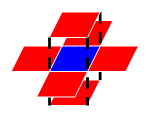## 题目

One important factor to identify acute stroke (急性脑卒中) is the volume of the stroke core. Given the results of image analysis in which the core regions are identified in each MRI slice, your job is to calculate the volume of the stroke core.

### Input Specification:

Each input file contains one test case. For each case, the first line contains 4 positive integers: M, N, L and T, where M and N are the sizes of each slice (i.e. pixels of a slice are in an M×N matrix, and the maximum resolution is 1286 by 128); L (≤60) is the number of slices of a brain; and T is the integer threshold (i.e. if the volume of a connected core is less than T, then that core must not be counted).

Then L slices are given. Each slice is represented by an M×N matrix of 0’s and 1’s, where 1 represents a pixel of stroke, and 0 means normal. Since the thickness of a slice is a constant, we only have to count the number of 1’s to obtain the volume. However, there might be several separated core regions in a brain, and only those with their volumes no less than T are counted. Two pixels are connected and hence belong to the same region if they share a common side, as shown by Figure 1 where all the 6 red pixels are connected to the blue one.Figure 1

### Output Specification:

For each case, output in a line the total volume of the stroke core.

### Sample Input:

 `````` 1 2 3 4 5 6 7 8 9 10 11 12 13 14 15 16 `````` ``````3 4 5 2 1 1 1 1 1 1 1 1 1 1 1 1 0 0 1 1 0 0 1 1 0 0 1 1 1 0 1 1 0 1 0 0 0 0 0 0 1 0 1 1 0 0 0 0 0 0 0 0 0 0 0 1 0 0 0 1 1 0 0 0 ``````

### Sample Output:

 ``````1 `````` ``````26 ``````

## 代码

 `````` 1 2 3 4 5 6 7 8 9 10 11 12 13 14 15 16 17 18 19 20 21 22 23 24 25 26 27 28 29 30 31 32 33 34 35 36 37 38 39 40 41 42 43 44 45 46 47 48 49 50 51 52 53 54 55 56 57 58 59 60 61 62 63 64 65 66 67 68 69 70 71 72 73 74 75 76 77 78 79 `````` ``````#include #include #include using namespace std; int dx[]={1,0,0,0,0,-1}; int dy[]={0,0,0,1,-1,0}; int dz[]={0,1,-1,0,0,0}; int m,n,l,t; //[x,y,z]=[m,n,l] int head; bool inq={false}; int count=0; struct node{ int x,y,z; }Node; bool judge(int x,int y,int z) { //boundary if(x>=m||x<0||y>=n||y<0||z>=l||z<0) return false; //state if(head[x][y][z]==0||inq[x][y][z]) return false; return true; } int bfs(int x,int y,int z) { int total=0; queue Q; Node.x=x; Node.y=y; Node.z=z; Q.push(Node); inq[x][y][z]=true; while(!Q.empty()) { node top=Q.front(); Q.pop(); total++; for(int i=0;i<6;i++) { if(judge(top.x+dx[i],top.y+dy[i],top.z+dz[i])) { Node.x=top.x+dx[i]; Node.y=top.y+dy[i]; Node.z=top.z+dz[i]; Q.push(Node); inq[top.x+dx[i]][top.y+dy[i]][top.z+dz[i]]=true; } } } if(total>=t) return total; else return 0; } int main() { scanf("%d%d%d%d",&m,&n,&l,&t); for(int i=0;i

## 分析

1. 遍历周围节点可以使用增量数组，xyz三个方向的增量参考图例就可以
2. 设置inq标志表示节点是否入过队，而非是否被访问过。如果使用是否被访问过作为标志，比如某个节点X当前已经在队列中，当前节点Y可以到达X，但X还未被访问，则发生X的重复入队
3. 统计某个相邻小区域的块数在bfs里增加total变量，每个节点pop出来计数一次，脑子里一直是DFS有点糊涂